# Precalculus : Solving Quadratic Equations

## Example Questions

1 3 Next →

### Example Question #7 : Find Roots Of Quadratic Equation Using Discriminant

Use the formula b2 - 4ac to find the discriminant of the following equation: x2 + 5x + 4 = 0.

Then state how many roots it has, and whether they are real or imaginary. Finally, use the quadratic function to find the exact roots of the equation.

Possible Answers:

Discriminant: 41

Two imaginary roots: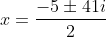Discriminant: 9

Two real roots: x = -1 or x = -4

Discriminant: 0

One real root: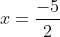Discriminant: 41

Two imaginary roots: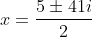Discriminant: 9

Two real roots: x = 1 or x = 4

Correct answer:

Discriminant: 9

Two real roots: x = -1 or x = -4

Explanation:

In the above equation, a = 1, b = 5, and c = 4. Therefore:

b2 - 4ac = (5)2 - 4(1)(4) = 25 - 16 = 9.

When the discriminant is greater than 0, there are two distinct real roots. When the discriminant is equal to 0, there is exactly one real root. When the discriminant is less than zero, there are no real roots, but there are exactly two distinct imaginary roots. In this case, we have two real roots.

Finally, we use the quadratic function to find these exact roots. The quadratic function is: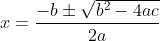Plugging in our values of a, b, and c, we get: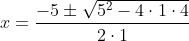This simplifies to: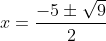which simplifies to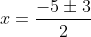which gives us two answers:

x = -1 or x = -4

These values of x are the two distinct real roots of the given equation.

### Example Question #8 : Find Roots Of Quadratic Equation Using Discriminant

Use the formula b2 - 4ac to find the discriminant of the following equation: -x2 + 3x - 3 = 0.

Then state how many roots it has, and whether they are real or imaginary. Finally, use the quadratic function to find the exact roots of the equation.

Possible Answers:

Discriminant: -21

Two imaginary roots: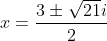Discriminant: -8

Two imaginary roots: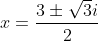.

Discriminant: 0

One real root: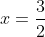Discriminant: -8

Two imaginary roots: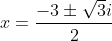Discriminant: -21

Two imaginary roots: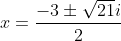Correct answer:

Discriminant: -8

Two imaginary roots:.

Explanation:

In the above equation, a = -1, b = 3, and c = -3. Therefore:

b2 - 4ac = (3)2 - 4(-1)(-3) = 9 - 12 = -3.

When the discriminant is greater than 0, there are two distinct real roots. When the discriminant is equal to 0, there is exactly one real root. When the discriminant is less than zero, there are no real roots, but there are exactly two distinct imaginary roots. In this case, we have two distinct imaginary roots.

Finally, we use the quadratic function to find these exact roots. The quadratic function is:Plugging in our values of a, b, and c, we get: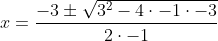This simplifies to: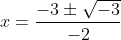Because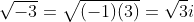, this simplifies to.  In other words, our two distinct imaginary roots are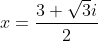and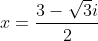### Example Question #9 : Find Roots Of Quadratic Equation Using Discriminant

Use the formula b2 - 4ac to find the discriminant of the following equation: x2 + 2x + 10 = 0.

Then state how many roots it has, and whether they are real or imaginary. Finally, use the quadratic function to find the exact roots of the equation.

Possible Answers:

Discriminant: -36

Two imaginary roots: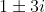Discriminant: 36

Two real roots: x = -5 or x = 7

Discriminant: -36

Two imaginary roots: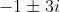Discriminant: 36

Two real roots: x = 5 or x = -7

Discriminant: 0

One real root: x = -1

Correct answer:

Discriminant: -36

Two imaginary roots:Explanation:

In the above equation, a = 1, b = 2, and c = 10. Therefore:

b2 - 4ac = (2)2 - 4(1)(10) = 4 - 40 = -36.

When the discriminant is greater than 0, there are two distinct real roots. When the discriminant is equal to 0, there is exactly one real root. When the discriminant is less than zero, there are no real roots, but there are exactly two distinct imaginary roots. In this case, we have two distinct imaginary roots.

Finally, we use the quadratic function to find these exact roots. The quadratic function is:Plugging in our values of a, b, and c, we get: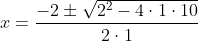This simplifies to: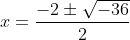Because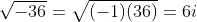, this simplifies to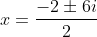. We can further simplify this to. In other words, our two distinct imaginary roots are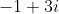and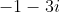.

### Example Question #10 : Find Roots Of Quadratic Equation Using Discriminant

Use the formula b2 - 4ac to find the discriminant of the following equation: x2 + 8x + 16 = 0.

Then state how many roots it has, and whether they are real or imaginary. Finally, use the quadratic function to find the exact roots of the equation.

Possible Answers:

Discriminant: 72

Two distinct real roots: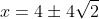Discriminant: 0

One real root: x = 4

Discriminant: 0

One real root: x = 0

Discriminant: 128

Two distinct real roots: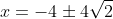Discriminant: 0

One real root: x = -4

Correct answer:

Discriminant: 0

One real root: x = -4

Explanation:

In the above equation, a = 1, b = 8, and c = 16. Therefore:

b2 - 4ac = (8)2 - 4(1)(16) = 64 - 64 = 0.

When the discriminant is greater than 0, there are two distinct real roots. When the discriminant is equal to 0, there is exactly one real root. When the discriminant is less than zero, there are no real roots, but there are exactly two distinct imaginary roots. In this case, there is exactly one real root.

Finally, we use the quadratic function to find these exact root. The quadratic formula is:Plugging in our values of a, b, and c, we get: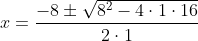This simplifies to: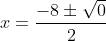which simplifies to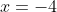This value of x is the one distinct real root of the given equation.

1 3 Next →

### All Precalculus Resources